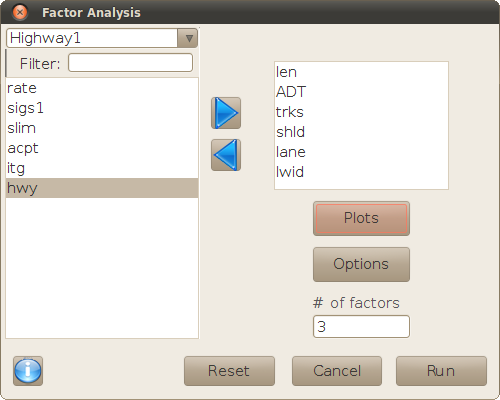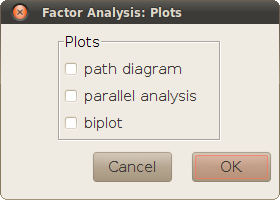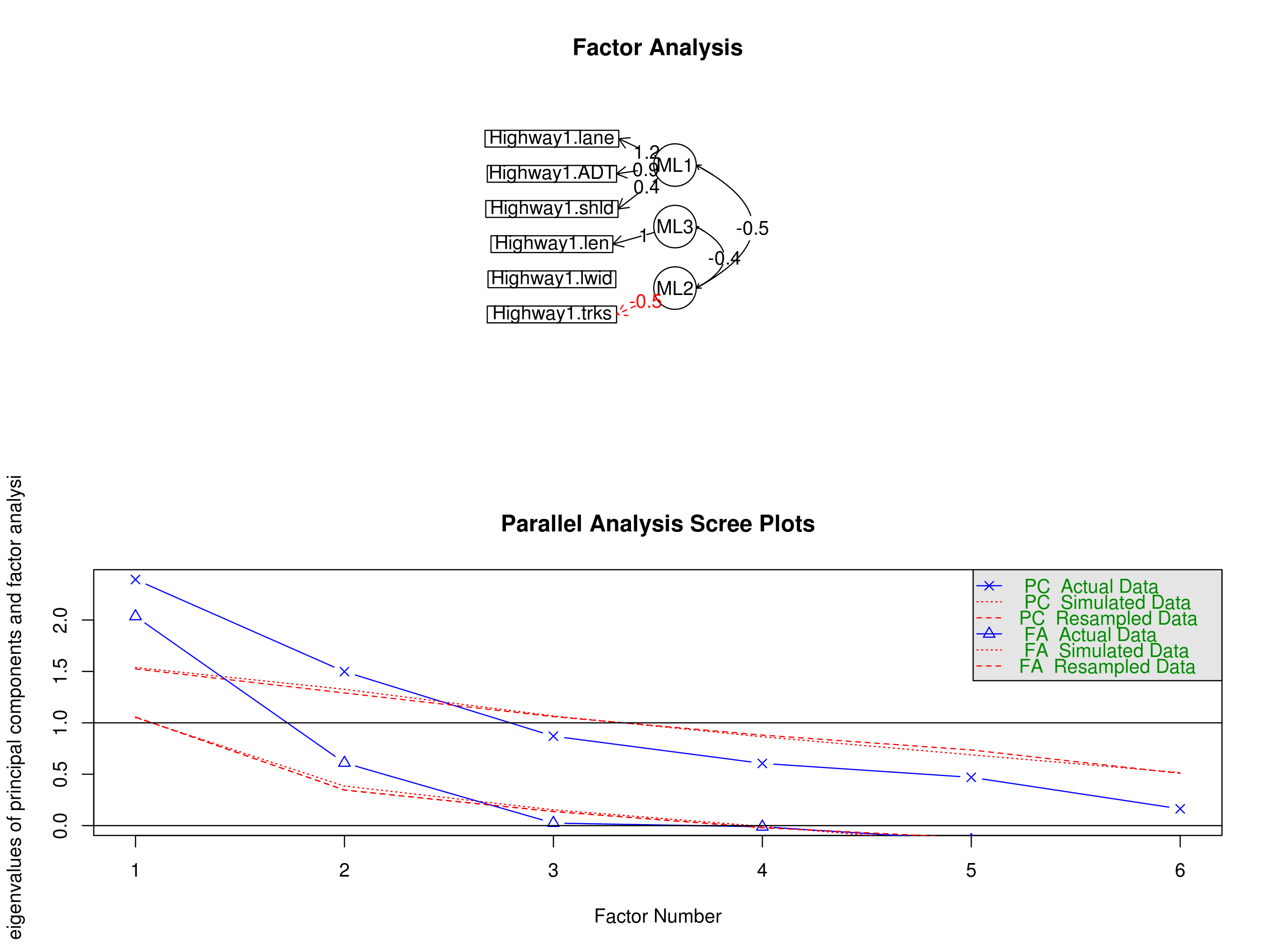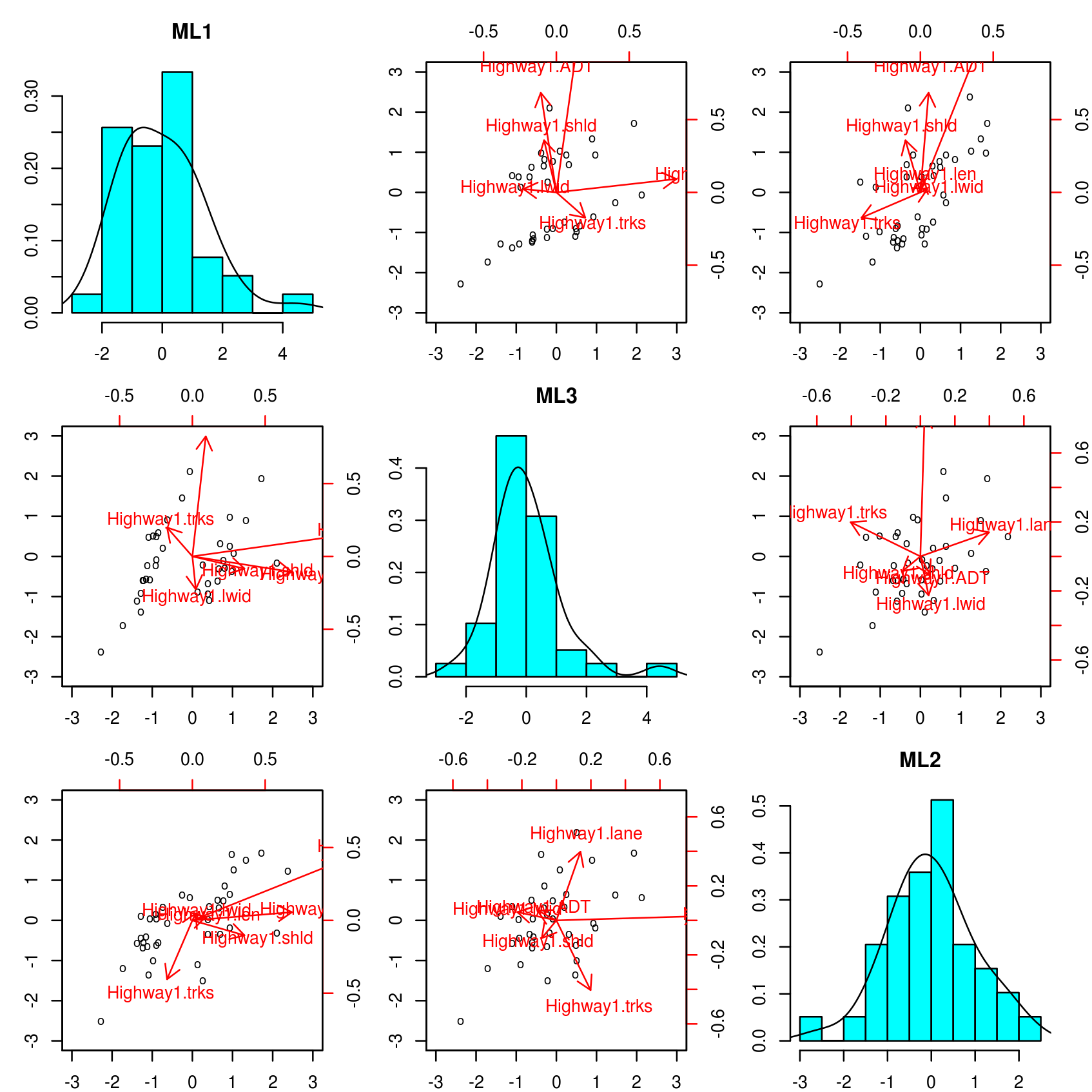## Description

Factor Analysis is used in experiments where n correlated variables are measured, and the researcher wants to extract a set of mn latent variables, that represent the variability of the original data as closely as possible.

These new variables are the so-called factors (nothing to do with the concept of a 'factor' variable in R), which are mutually independent, linear transformations of the original variables. The linear relation between both sets of variables is represented by a matrix of nm coefficients or loadings. For each possible combination of the original variables, this matrix determines a projection in the sub-space spanned by the factors, and the objective of the analysis is to achieve minimal differences between the original observations and their projections in that sub-space.

Any scaling of each factor by a constant would represent an equivalent solution to the problem. In order to standardize the results, the loadings matrix contains the Pearson correlations between factors and variables. This is an univocal scaling, where loadings are always in the range [-1, 1], and their sums of squares along each factor is proportional to the amount of variance explained by that factor.

Note: This dialog uses the functions `fa` and `principal` of package psych, not `factanal` from the standard stats package. Notice that the results of `principal` are those of a factor analysis using the extraction method of principal components, not the results of a principal components analysis (like `princomp` or `prcomp`). See more information on these methods in the following links:

## Variable selection

The main dialog is used to select the original variables from a data frame of the workspace, and the number of factors that will be defined. This is an arbitrary number chosen by the researcher, although parts of the results in a first trial may be used to redefine the model with a different number of factors.There also are two buttons for plotting some results and setting some options of the analysis, as explained in the following sections.

## Options

This sub-dialog is used for setting the options of the analysis:• Matrix: use the correlations or the variances-covariances between variables as the input for the analysis. The correlation matrix (chosen by default) standardizes the relations between the original variables, removing the possible effects of using different scales across variables.
• Extraction method: there are several available methods for evaluating the 'proximity' between the observed variables and their projections, and selecting the set of factors that optimize it. This dialog sets 'maximum likelihood' as default choice, which is the default of `factanal` as well (but not the default of `fa`).
• Rotation: in a first instance, a factor analysis attempts to explain as much variance of the observed data as possible, with as few factors as possible. But researchers usually want the factors to be 'meaningful' in terms of the original variables as well, i.e. obtain factors highly correlated with a few variables, and little correlated with the rest. After extracting a set of factors, the method chosen in this option 'rotates' them, so that the matrix of loadings is changed to meet this criterion, but the sub-space spanned by the new factors is exactly the same as for the non-rotated factors.
• Sorted by size: factors are always sorted by explained variance in descending order, but the original variables are sorted in the loadings matrix by the arbitrary order whereby they were introduced in the variable selection. This option sorts the variables by the absolute value of their loadings for the first factor, and then for the second, etc., in descending order.
• Cut loadings less than...: hide the values of loadings below an arbitrary absolute value, in order to highlight the strongest correlations between factors and original variables (this only changes the presentation of the loadings matrix, it does not change the results).
• Save scores: each observation has a 'score' associated to each factor; the set of m scores define the projection of the observation in the sub-space spanned by the factors, and may be used for subsequent analyses. This option creates a data frame named `X.1` (where `X` stands for the name of the original data frame), that contains the n original variables selected for the analysis, plus the m scores.

## Plots

This sub-dialog contains a list of check boxes for representing different plots:### Path diagram

A visual representation of the correlations between original variables and factors: the former are drawn in rectangles, and the latter in circles. The variables and factors that have a correlation greater than 0.3 are graphically connected between them (this is the same information conveyed by the loadings matrix if the values are cut below 0.3). The correlations between different factors are drawn as well.

### Parallel analysis

When there is no special reason for deciding the number of latent factors, researchers often take a decision based on the amount of variance explained by the first factors (ordered by explanatory power), and the gain obtained for additional factors, as seen in 'scree plots'. The parallel diagram prints such plots for the observed data and artificial, random uncorrelated data used as baseline. The crossing between these scree plots may be used for 'suggesting' number of factors, as the maximum number that explains an amount of variance of the actual data proportionally greater than for the artificial data (before both scree plots cross). The plots are printed for two representative methods of factor extraction (principal components and principal axis factoring), regardless of the method actually set in Options (see below). If the path diagram is also selected, the parallel analysis is shown below in the same figure.### Biplot

This plot represents a matrix of scatterplots and histograms, with the projections of the observed data in different planes of the sub-space spanned by the extracted factors. This may be used to see how the observations are distributed along the scale of the different factors. The superimposed arrows are projections of the original variables in those planes, defined from the vectors (columns) of the loadings matrix; the longer and more parallel to the horizontal or vertical axis, the stronger will be the relation between the represented variable and the factor corresponding to that axis. This essentially is what the path diagram represents as well, although the information of the biplots is more comprehensive (and also more difficult to grasp if the number of factors is greater than two or three).